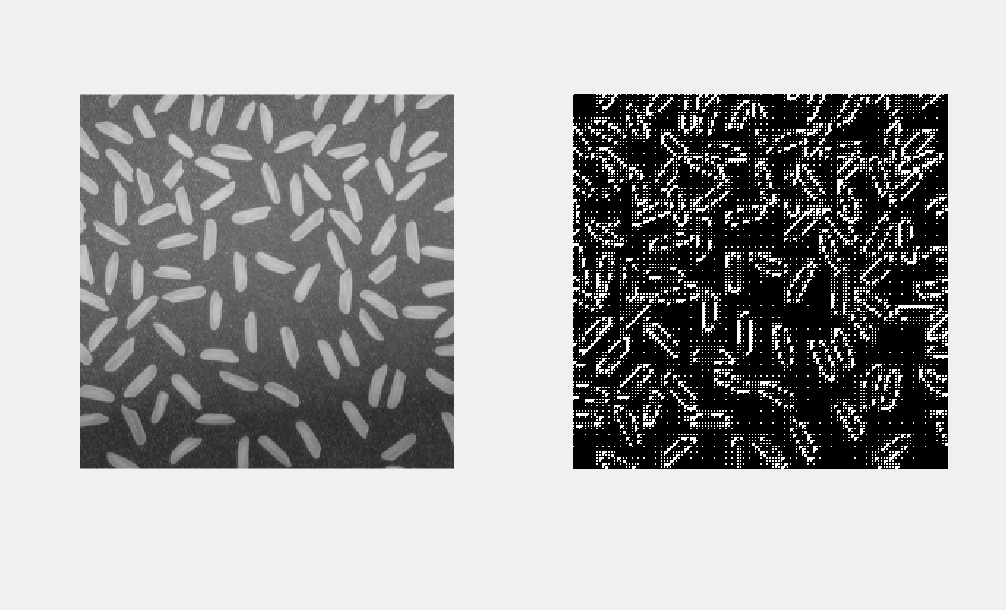# 阈值分割与区域分割

Posted by jjx on January 1, 2017

• 阈值分割技术
• 基于区域的图像分割技术
• 本章的典型案例
• 基于LoG和Canny算子的精确边缘检测
• 基于Hough变换的直线检测
• 图像的四叉树分解

### 阈值分割

• 确定阈值．
• 将阈值和像素比较，．
• 把像素归类

#### 阈值分割方法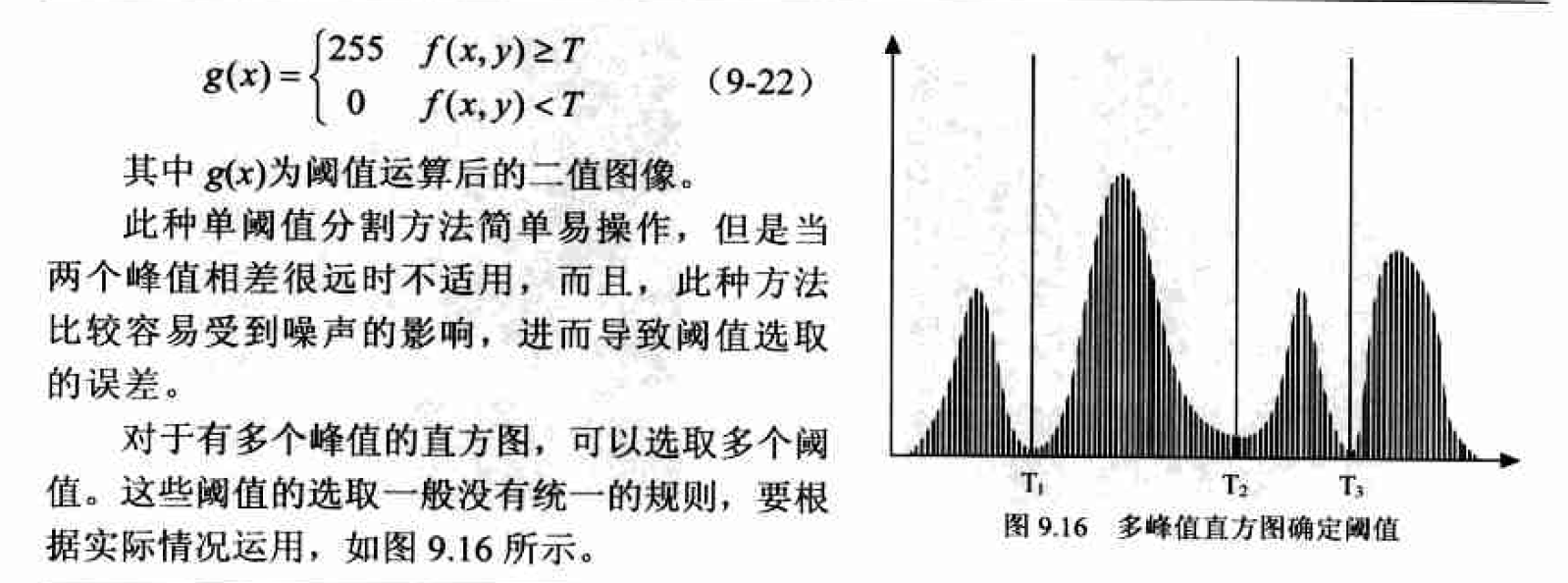(1)选择一个T的初始估计值。
(2)利用阈值T把图像分为两个区域R1, 和R2
(3)对区域R1和R2中的所有像素计算平均灰度值μ1和μ2
(4)计算新的阈值：
$T=\frac{1}{2}(u_1+u_2)$
(5)重复步骤2-4, 直到逐次迭代所得的T值小于事先定义的参数T。

$p(z) = P_1p_1(z) + P_2p_2(z)$

$P_1+P_2=1$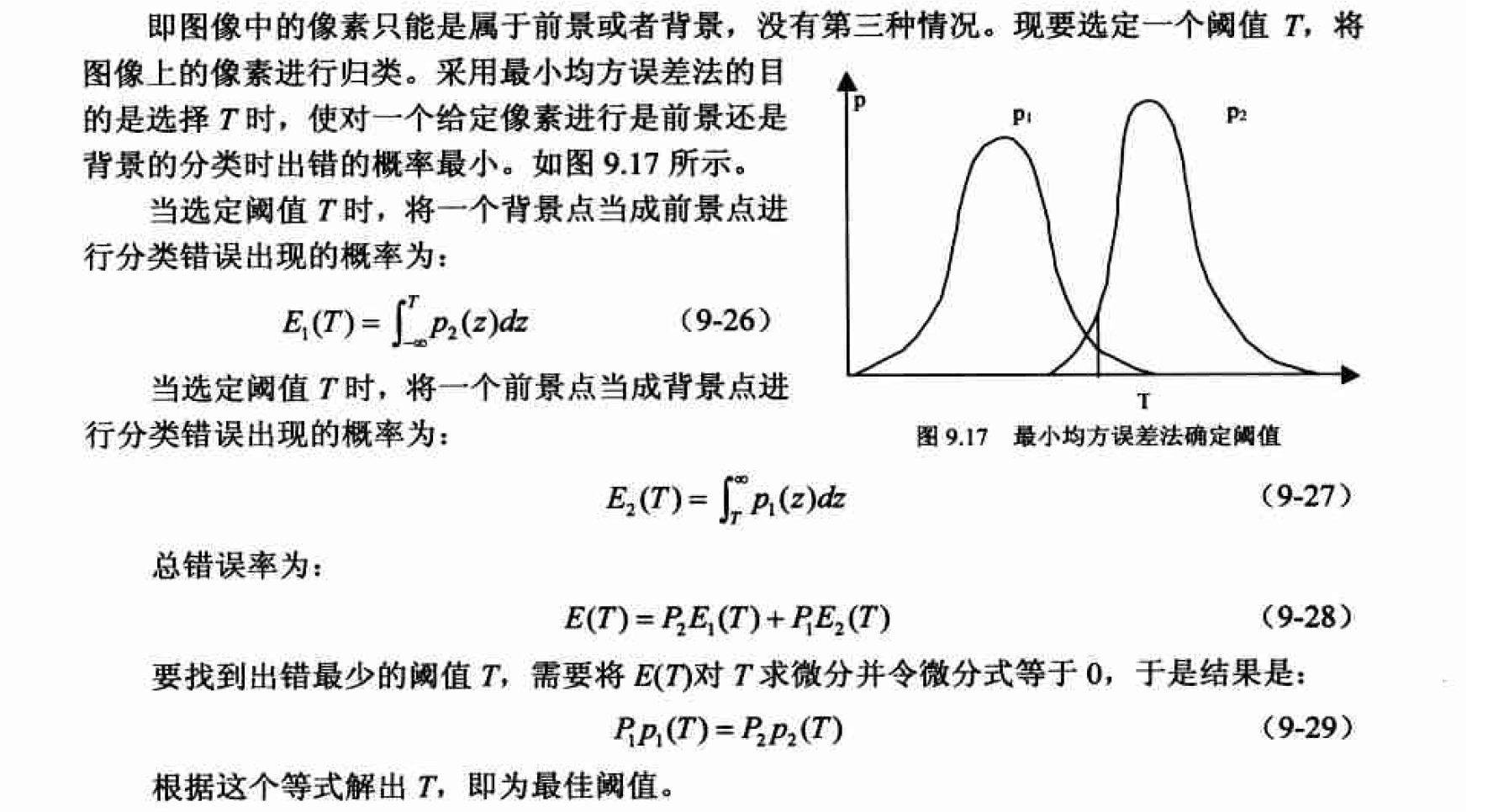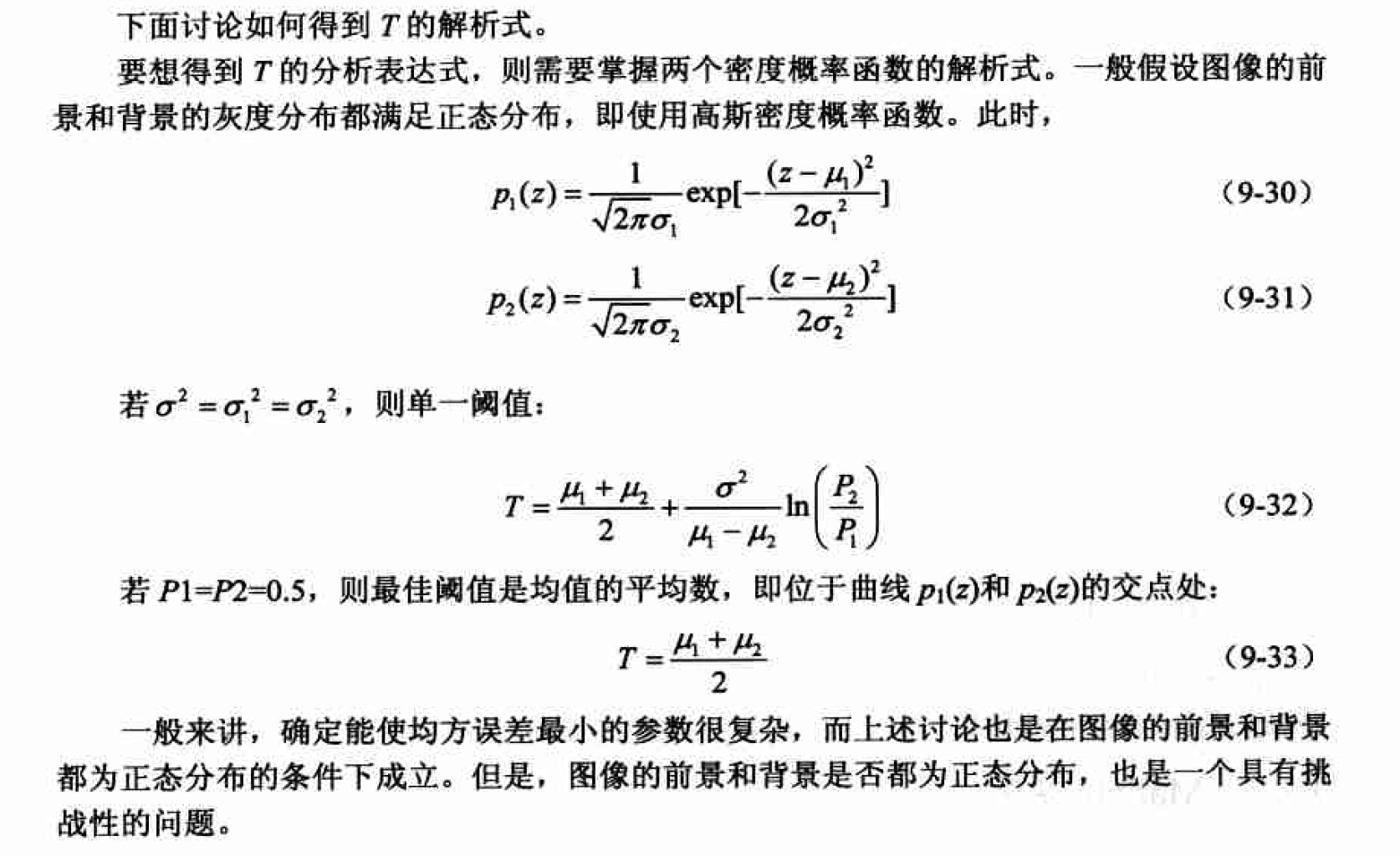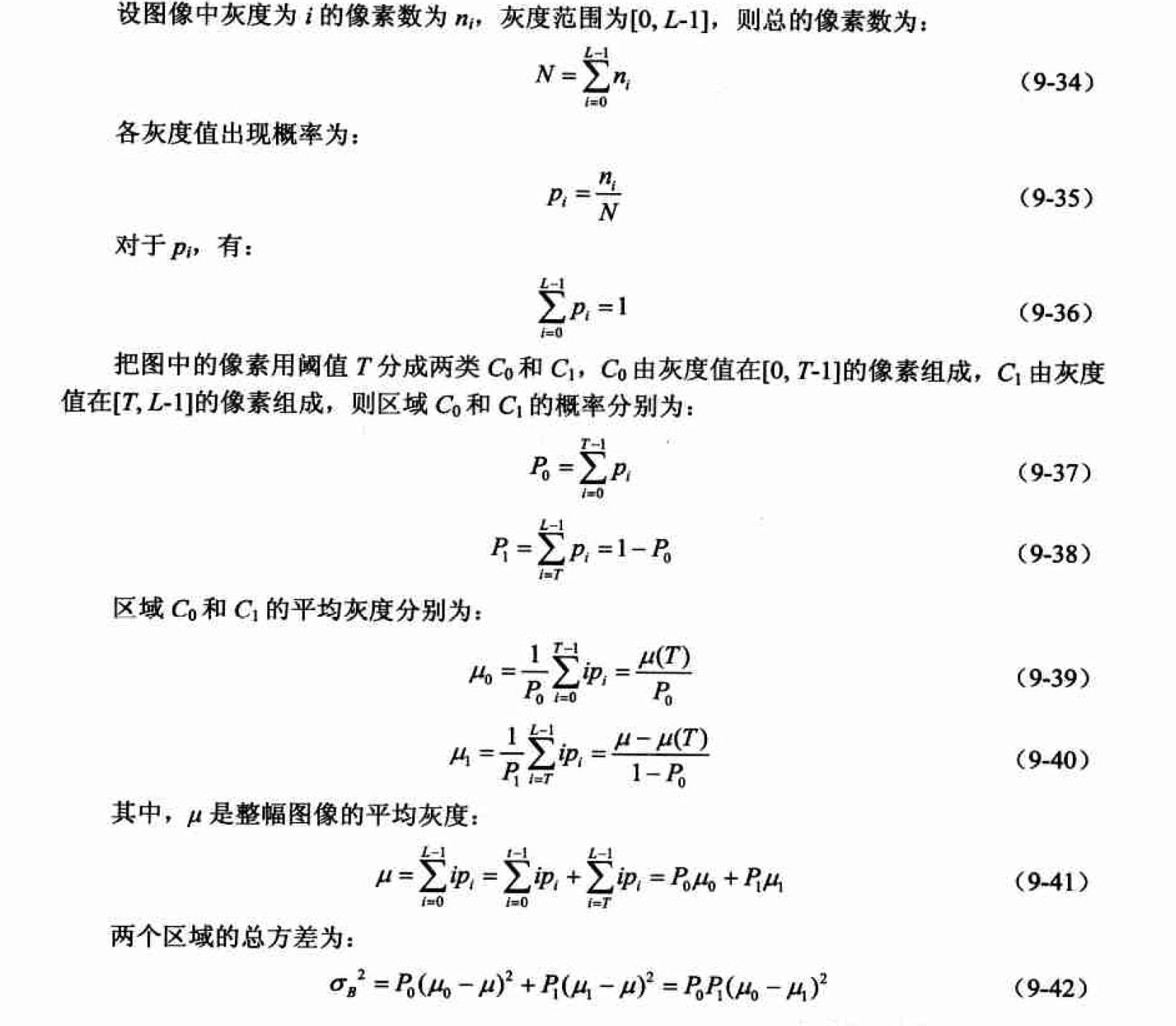#### Matlab实现

Matlab中和阙值变换相关的两个主要函数是im2bw和graythresh。实际上，利用graythresh函数即可实现最大类间方差法。

function [Ibw, thres] = autothreshold(I)
% 迭代法自动阈值分割
%
% 输入：I - 要进行自动阈值分割的灰度图像
% 输出：Ibw - 分割后的二值图像
%      thres - 自动分割采用的阈值

thres = 0.5 * (double(min(I(:))) + double(max(I(:)))); %初始阈值
done = false; %结束标志
while ~done
g = I >= thres;
Tnext = 0.5 * (mean(I(g)) + mean(I(~g)));
done = abs(thres - Tnext) < 0.5;
thres = Tnext;
end;

Ibw = im2bw(I, thres/255); % 二值化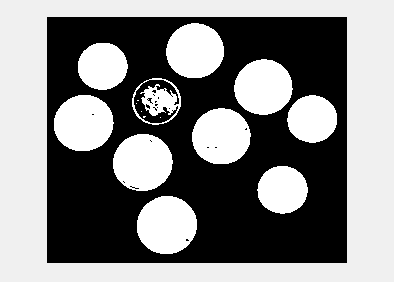### 区域分割

#### 区域生长及其实现

(1)选择合适的生长点。
(2)确定相似性准则即生长准则。
(3)确定生长停止条件。

matlab实现

function J = regionGrow(I)
% 区域生长，需要以交互方式设定初始种子点，具体方法为鼠标单击图像中一点后，按下回车键
%
% 输入：I - 原图像
% 输出：J - 输出图像

if isinteger(I)
I=im2double(I);
end
figure,imshow(I),title('原始图像')
[M,N]=size(I);
[y,x]=getpts;             %获得区域生长起始点
x1=round(x);            %横坐标取整
y1=round(y);            %纵坐标取整
seed=I(x1,y1);           %将生长起始点灰度值存入seed中
J=zeros(M,N);          %作一个全零与原图像等大的图像矩阵J，作为输出图像矩阵
J(x1,y1)=1;             %将J中与所取点相对应位置的点设置为白
sum=seed;              %储存符合区域生长条件的点的灰度值的和
suit=1;                 %储存符合区域生长条件的点的个数
count=1;               %记录每次判断一点周围八点符合条件的新点的数目
threshold=0.15;         %阈值，注意需要和double类型存储的图像相符合
while count>0
s=0;                   %记录判断一点周围八点时，符合条件的新点的灰度值之和
count=0;
for i=1:M
for j=1:N
if J(i,j)==1
if (i-1)>0 & (i+1)<(M+1) & (j-1)>0 & (j+1)<(N+1)  %判断此点是否为图像边界上的点
for u= -1:1                               %判断点周围八点是否符合阈值条件
for v= -1:1
if  J(i+u,j+v)==0 & abs(I(i+u,j+v)-seed)<=threshold& 1/(1+1/15*abs(I(i+u,j+v)-seed))>0.8
J(i+u,j+v)=1;
%判断是否尚未标记，并且为符合阈值条件的点
%符合以上两条件即将其在J中与之位置对应的点设置为白
count=count+1;
s=s+I(i+u,j+v);                      %此点的灰度之加入s中
end
end
end
end
end
end
end
suit=suit+count;                                   %将n加入符合点数计数器中
sum=sum+s;                                     %将s加入符合点的灰度值总合中
seed=sum/suit;                                    %计算新的灰度平均值
end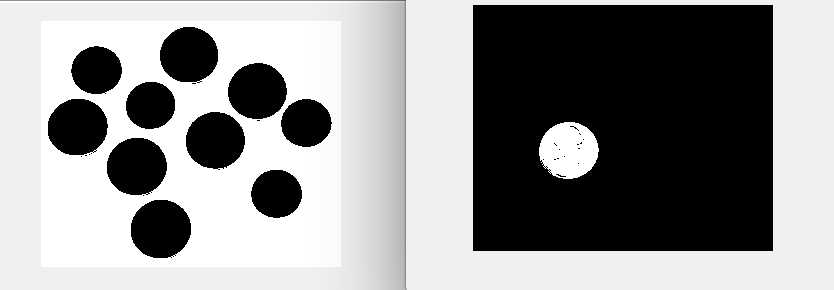选择不同的生长点，结果不同。

#### 区域分裂与合并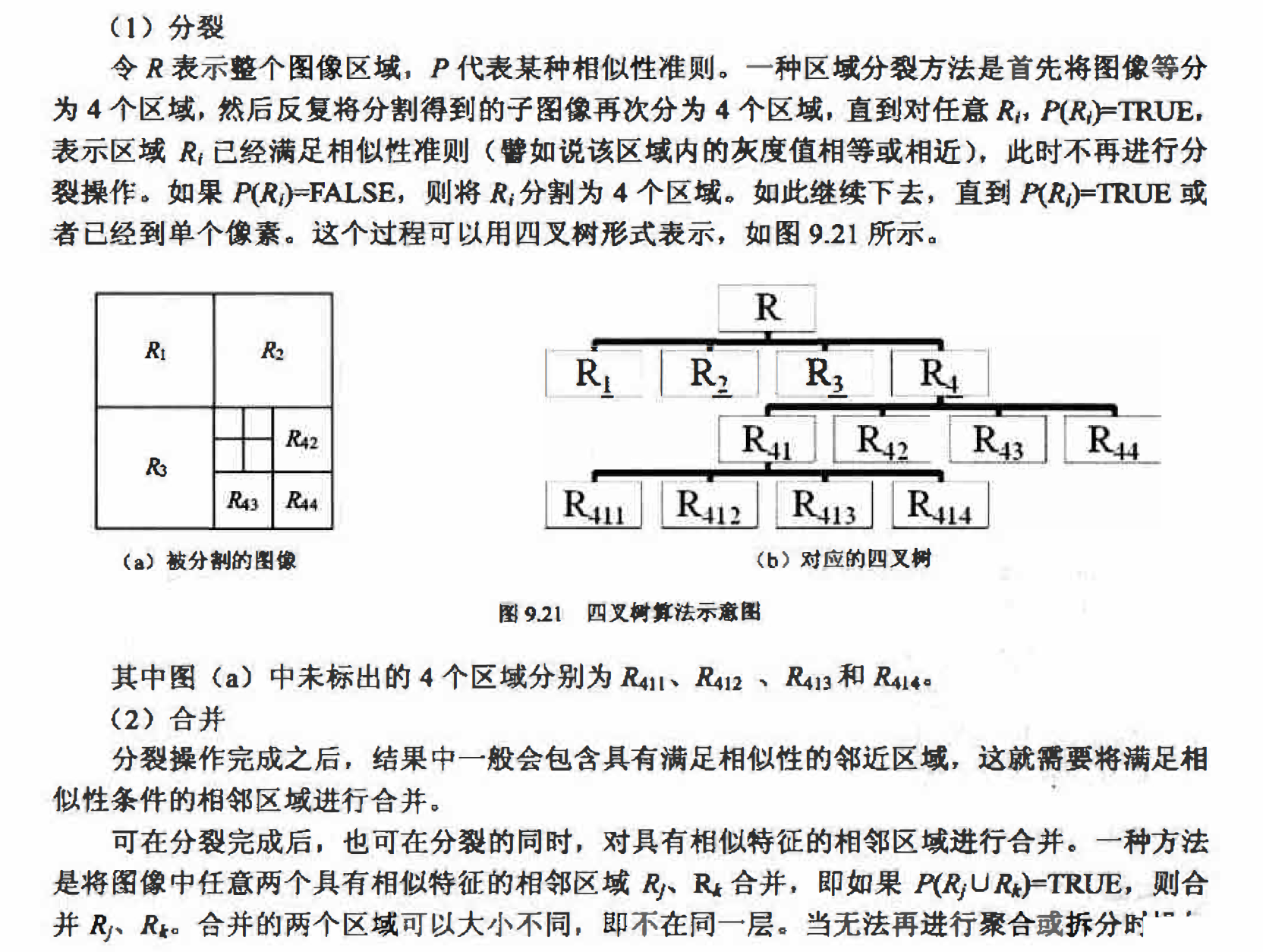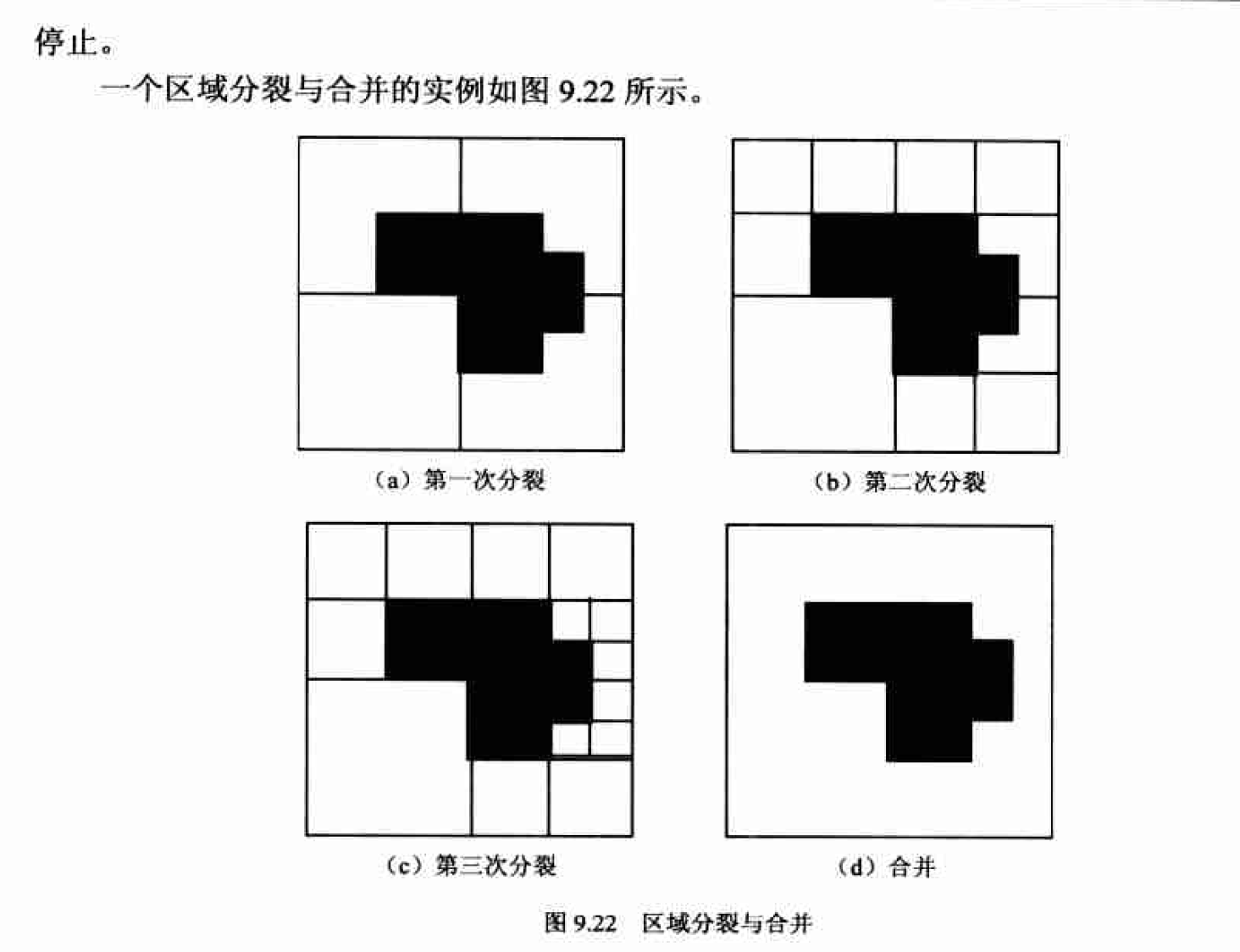图像先分裂为如图9.22Ca)所示；第二次分裂时，如图(b)所示，由于左下角区域满足$P(R_i)=TRUE$,则不进行分裂操作；第三次分裂时，如图(c)所示，仅仅右边的突出部分 $P(R_i)=FALSE$, 需要进行分裂操作，其余不变，完成后，分裂停止；最后，对两个相邻区域 实行合并，一直得到最后的结果，如图(d)所示。

(1) qtdecomp函数
Matlab的IPT函数qtdecomp可以进行四叉树分解。该函数首先将图像划分成相等大4的4块，然后对每一个块进行一致性检查。如果该块不符合一致性标准， 则将该块继续分为4块； 否则不对其进行进一步的分割。这个过程将会一直重复直至每一个块都符合一致性标 准， 分解的结果可能会包含许多大小不同的块。
qtdecomp函数的常用调用形式为：
S = qtdecomp(I,threshold,[mindim,maxdim])

threshold是分割成的子块中允许的阈值，默认值为0.如杲子块中最大元素和最小元 素的差值小于该阈值就认为满足一致性条件．对于double型矩阵，threshold将直接作为阈值；而对于uinit8和uintl6类型的矩阵，threshold将被乘以255和65535以作为实际阈值．对于图像而言，threshold的取值范围是0到1.

[mindim maxdim］是尺度阈值.mindim参数可以屏蔽函数对尺度上小于mindim的子块的处理，而不论这个子块是否满足一致性条件；如果参数形式为[mindim maxdim], 则表示不产生小于mindim尺度的子块，也不保留大于maxdim尺度的子块，此时 maxdim/mindim必须是2的整数次幂

(2) qtgetblk函数 在得到稀疏矩阵S后， 利用IPT函数qtgetblk可进一步获得四叉树分解后所有指定大小的子块像素及位置信息。常用调用形式为：
[vals,r,c]=qtgetblk(I,S,dim)

dim是指定子块的大小

vals是dimdimk的三维矩阵，包含I中所有符合条件的子块数据。其中k为符合 条件的dim*dim的大小的子块的个数，vals(:.:,i)表示符合条件的第i个子块的内容．
r和c均为列向量，分别表示图像I中符合条件子块左上角的纵坐标（行索引）和横坐标（列索引）．

(3) qtsetblk函数

J = qtsetblk(I,S,dim,vals)
S是I经过qtdecomp函数处理的结果．
dim是指定的子块大小．
vals是dimdimk的三维矩阵，包含了用来替换原有子块的新子块信息．其中K应为图像I中大小为dim*dim的子块的总数，vals(:,:,i)表示要替换的第i个子块．

I1 = imread('rice.png');

S = qtdecomp(I1,0.2);
S2 = full(S);

figure;
subplot(1,2,1),imshow(I1);
subplot(1,2,2),imshow(S2);

ct = zeros(6,1);
for ii = 1:6
[vals{ii},r,c]=qtgetblk(I1,S2,2^(ii-1));
ct(ii) = size(vals(ii),3);
end